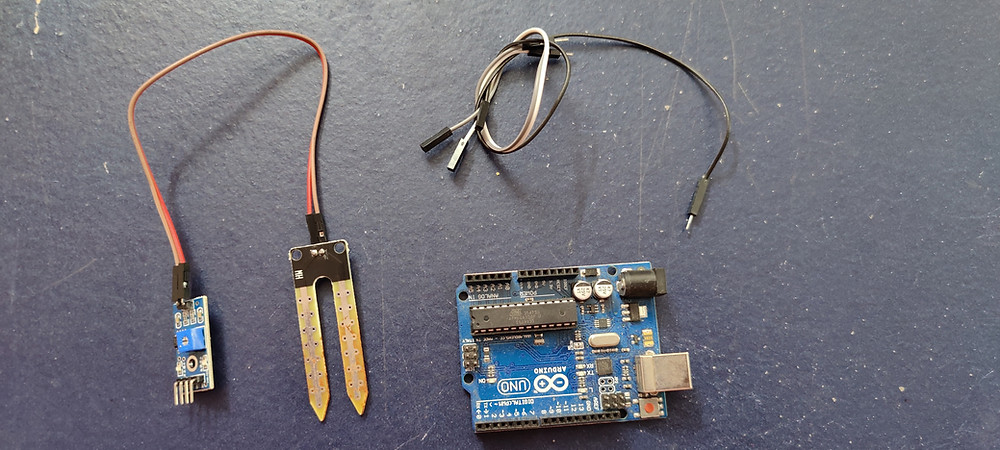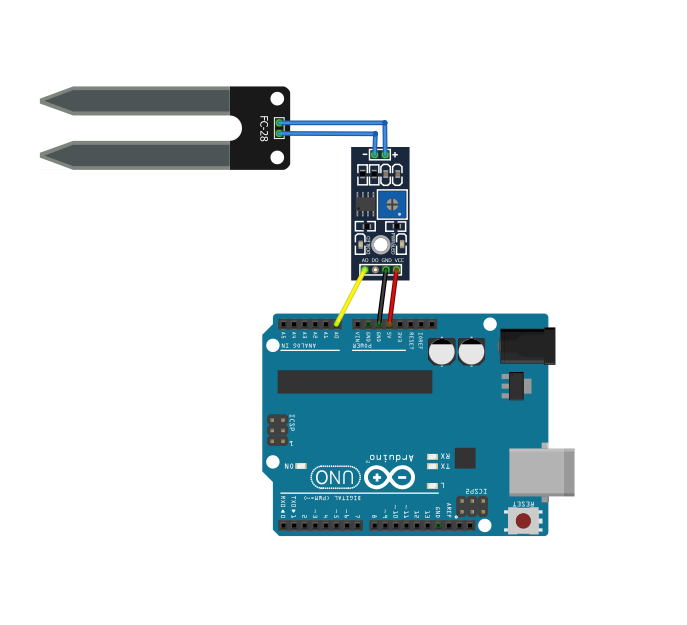• nithya7rns

# Interfacing Soil/Moisture Sensor with Arduino

Updated: Dec 31, 2020Soil Moisture Sensor

The quantity of water present in the soil is the #soil moisture. The moisture of the soil is measured to determine how fertile the land is to cultivate a particular crop on a large scale. Basically, #moisture in the soil is an indirect measure of properties such as its dielectric constant, interaction with neutrons, etc.

In this project, we are going to design a circuit using a Soil Moisture sensor to test the moisture quantity in Soil with different textures. This article gives the Hardware description and explains the circuit connection and the code required to interface with the IDE to get the output on the computer screen.

## Hardware List

The circuit consists of the following components.All the Components Required
1. ArduinoArduino UNO

Arduino board is a microcontroller that is used to accept inputs from sensors connected and provide an output action on the desired device connected to it. The sensor inputs can be that from light-detecting sensors, flame sensors, temperature sensors, etc. The output function done on the devices can be turning on an LED, the sound of a buzzer, or a Serial monitor display.

2. Soil Moisture sensorFC-28 Soil Moisture Sensor

The sensor (also called a Hygrometer #sensor) is used to measure the volumetric quantity of water in the soil through the probes provided. The FC-28 sensor contains an IC on the board which is an LM323 comparator. The sensor also contains a #potentiometer embedded in it which will hold the threshold value of the moisture set by the user.

Sensor specifications.

• Input voltage: 3.3V - 5V

• Output voltage: 0V - 4.2V

• Input current: 35 mAmps

• Output: Analog or Digital (Analog Range 0 - 1023 and Digital outputs 0 or 1)

3. Jumper WiresJumper Wires

These are the main components that are used to establish the connections between different devices of the circuit.

## Circuit Diagram

The circuit diagram below shows the necessary connections from the Arduino to the FC-28 Soil Moisture Sensor.Circuit Connections

The Soil Moisture sensor is connected to the Arduino board with the help of Jumper wires. The sensor contains a set of pins on two sides. The set of pins on one side are Ground(Gnd), #Voltage input(Vcc), Digital output(D0), and Analog output(A0).

These pins are connected to the Arduino chip in the following manner. The Ground pin of the Sensor is connected to the Ground pin of the #Arduino chip. The Vcc pin on the sensor is connected to the 5V supply pin of the Arduino chip.

When there is a requirement for Analog output, the A0 pin of the sensor is connected to the A0 pin of the Arduino chip. The range of output values for Analog output is 0 - 1023. Else, when Digital output is required, the D0 pin of the sensor can be connected to any of the digital output pins of the Arduino chip. The digital outputs as we know will be either 0 or 1.

The second set of pins present on the other side of the sensor are connected to the probes that will be measuring the moisture of the soil when placed physically in it. The conducting probes are the basic sensor devices of this circuit.

As mentioned earlier, the sensor has a potentiometer and a #comparator embedded on it whose functions are to hold the threshold value and compare the input value from the sensor to the threshold value fixed by the user respectively. The detailed working will be discussed in the working of the circuit.

### Code:

The following code needs to be entered into the Arduino IDE and injected into the chip.

`const int moist_pin = A0;`

In the beginning, a constant integer value is declared for the Analog pin of the Arduino chip (The sensor A0 pin is connected to it). The name given to this integer here is moist_pin. This will be holding the values of input from the sensor.

```void setup() {
Serial.begin(9600);
}```

This is the setup( ) function present in all the codes that are required to run on the Arduino chip. Here a Baudrate of 9600 is given for the Serial.begin() statement. Any other desired value can be given as the Baudrate based on the requirement.

```void loop() {
float moisture;
int op;```

The loop( ) function starts after the setup of the device is done. The loop function continues to run the program until a specific condition is given to stop the execution of the code. So, in the loop( ) function, two variables, namely, "moisture" which is a floating-point number, and "op" which is an integer are declared.

`op = analogRead(moist_pin);`

The variable "op" is assigned to take in the value from a function called analogRead( ) which takes in the input value from the sensor. The value measured by the sensor is stored in moist_pin. This value is given as input to the analogRead( ) function which reads the value and further assigns it to the variable "op" as per the above statement.

`moisture = ( 100 - ( (op/1023.00) * 100 ) );`

This is the main conversion statement required for this code to work properly to get the desired result. The floating-point variable "moisture" declared is used to store the input value after the conversion. "op" which is the sensor input value is divided by 1023.00 (to convert into decimal value as it is declared as an integer) and multiplied by a factor of 100 (to convert into percentage value).

Now, "( (op/1023.00) * 100 )" gives the value of dryness of the soil. Subtracting the value of dryness from a total of 100% gives the value of moisture present in the soil. The above-given statement does the conversion and stores the value of moisture content in the soil in the variable "moisture".

`Serial.print("Moisture Percentage = ");`

The Serial.print( ) statement is used for the output to be displayed on the Serial Monitor. Here through this statement, it displays a sentence "Moisture Percentage = ".

`Serial.print(moisture);`

The next output statement is used to display the value of the "moisture" variable which stores the input value from the sensor after the required conversion is done.

`Serial.print("%\n\n");`

This output statement is for formatting which uses an Escape sequence "\n" twice to display the next output after two lines.

`delay(1000);`

A delay of one second is given in between the consecutive outputs.

COMPLETE CODE

```const int moist_pin = A0;

void setup() {
Serial.begin(9600);
}

void loop() {
float moisture;
int op;
moisture = ( 100 - ( (op/1023.00) * 100 ) );
Serial.print("Moisture Percentage = ");
Serial.print(moisture);
Serial.print("%\n\n");
delay(1000);
}```

code
.txt
TXT • 311B

Note: Please change the file format to .ino(Arduino IDE) format before you upload the code to the Arduino board.

### Working:

The circuit, when switched ON and OFF, is shown below.Circuit switched OFF

When the connections are done, the conducting probes connected to the Soil moisture sensor must be inserted into the soil whose Moisture value is desired to be known. The circuit must simultaneously be interfaced with Arduino IDE.Circuit switched ON

When the circuit is switched ON, the sensor reads the values that the probes conduct. The potentiometer actually holds the threshold value fixed by the user. The LM323 comparator present on the sensor chip compares the threshold value to the sensor input value. The glowing of the LED in the sensor indicates the functioning of the sensor.

(In the code provided above, the threshold value is not given and hence no comparison happens as it is the function which we can code/design as per our requirement).

Finally, the output, that is the value of moisture content in the soil is displayed on the Serial Monitor of the Arduino IDE.

The video given below shows an explained version of the circuit building, working of the code, and display of output for better understanding.

Video By - Abhishek Goud

The article gives the idea for basic connections of the Soil Moisture Sensor to Arduino and how to read the values. This sensor and circuit can be used as Moisture measurement devices for gardens at home or for agricultural fields on developing the same on a larger scale.